## A force on a particle depends on position such that F(x) = (3.00 N/m2)x2 + (6.00 N/m)x for a particle constrained to move along the x-axis.

Question

A force on a particle depends on position such that F(x) = (3.00 N/m2)x2 + (6.00 N/m)x for a particle constrained to move along the x-axis. What work is done by this force on a particle that moves from x = 0.00 m to x = 2.00 m?

in progress 0
2 weeks 2021-08-29T02:58:31+00:00 2 Answers 0 views 0

The work done by a particle from x = 0 to x = 2 m is 20 J.

Explanation:

A force on a particle depends on position constrained to move along the x-axis, is given by,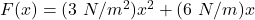We need to find the work done on a particle that moves from x = 0.00 m to x = 2.00 m.

We know that the work done by a particle is given by the formula as follows :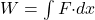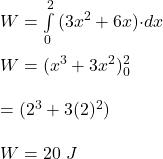So, the work done by a particle from x = 0 to x = 2 m is 20 J. Hence, this is the required solution.

Explanation:

Force is given by

F = 3x² + 6x

Particle moves from x = 0 m to 2 m

Work done is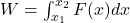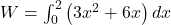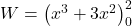W = 8 + 12 – 0 – 0

W = 20 J